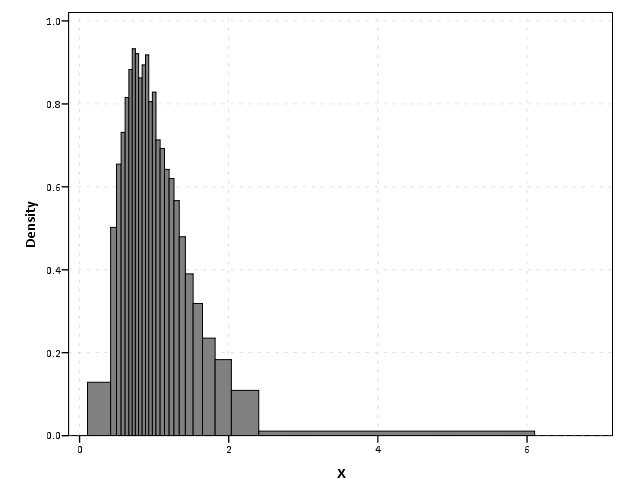# SPSS Statistics

View Only

### Equal Probability Histograms in SPSS

#### By Archive User posted Wed October 23, 2013 10:43 AM

The other day on NABBLE an individual asked for displaying histograms with unequal bar widths. I showed there if you have the fences (and the height of the bar) you can draw the polygons in inline GPL using a `polygon` element and the `link.hull` option for edges. I used a similar trick for spineplots.

On researching when someone would use unequal bar widths a common use is to make the fences at specified quantiles and plot the density of the distribution. That is the area of the bars in the plot is equal, but the width varies giving the bars unequal height. Nick Cox has an awesome article about graphing univariate distributions in Stata with equally awesome discussion of said equal probability histograms.

The full code is at the end of the post, but in a nutshell you can call the `!EqProbHist` MACRO by specifying the `Var` and how many quantiles to slice it, `NTiles`. The macro just uses OMS to capture the table of NTiles produced by `FREQUENCIES` along with the min and max, and returns a dataset named `FreqPoly` with the lower and upper fences plus the height of the bar. This dataset can then be plotted with a seperate `GGRAPH` command.

``!EqProbHist Var = X NTiles = 25.GGRAPH  /GRAPHDATASET DATASET = 'FreqPoly' NAME="graphdataset" VARIABLES=FenceL FenceU Height  /GRAPHSPEC SOURCE=INLINE.BEGIN GPL SOURCE: s=userSource(id("graphdataset")) DATA: FenceL=col(source(s), name("FenceL")) DATA: FenceU=col(source(s), name("FenceU")) DATA: Height=col(source(s), name("Height")) TRANS: base=eval(0) TRANS: casenum = index()  GUIDE: axis(dim(1), label("X")) GUIDE: axis(dim(2), label("Density")) SCALE: linear(dim(2), include(0)) ELEMENT: polygon(position(link.hull((FenceL + FenceU)*(base + Height))), color.interior(color.grey), split(casenum)) END GPL.``

An example histogram is below.Note if you have quantiles that are tied (e.g you have categorical or low count data) you will get division by zero errors. So this type of chart is only reasonable with continuous data.

``*********************************************************************************************.*Defining Equal Probability Macro - only takes variable and number of tiles to slice the data.DEFINE !EqProbHist (Var = !TOKENS(1)                   /NTiles = !TOKENS(1) )DATASET DECLARE FreqPoly.OMS/SELECT TABLES/IF SUBTYPES = 'Statistics'/DESITINATION FORMAT = SAV OUTFILE = 'FreqPoly' VIEWER = NO.FREQUENCIES VARIABLES=!Var  /NTILES = !NTiles  /FORMAT = NOTABLE  /STATISTICS = MIN MAX.OMSEND.DATASET ACTIVATE FreqPoly.SELECT IF Var1 <> "N".SORT CASES BY Var4.COMPUTE FenceL = LAG(Var4).RENAME VARIABLES (Var4 = FenceU).COMPUTE Height = (1/!NTiles)/(FenceU - FenceL).MATCH FILES FILE = */KEEP FenceL FenceU Height.SELECT IF MISSING(FenceL) = 0.!ENDDEFINE.*Example Using the MACRO and then making the graph.dataset close all.output close all.set seed 10.input program.loop #i = 1 to 10000.  compute X = RV.LNORMAL(1,0.5).  compute X2 = RV.POISSON(3).  end case.end loop.end file.end input program.dataset name sim.PRESERVE.SET MPRINT OFF.!EqProbHist Var = X NTiles = 25.GGRAPH  /GRAPHDATASET DATASET = 'FreqPoly' NAME="graphdataset" VARIABLES=FenceL FenceU Height  /GRAPHSPEC SOURCE=INLINE.BEGIN GPL SOURCE: s=userSource(id("graphdataset")) DATA: FenceL=col(source(s), name("FenceL")) DATA: FenceU=col(source(s), name("FenceU")) DATA: Height=col(source(s), name("Height")) TRANS: base=eval(0) TRANS: casenum = index()  GUIDE: axis(dim(1), label("X")) GUIDE: axis(dim(2), label("Density")) SCALE: linear(dim(2), include(0)) ELEMENT: polygon(position(link.hull((FenceL + FenceU)*(base + Height))), color.interior(color.grey), split(casenum)) END GPL.RESTORE.*********************************************************************************************.``

#datavisualization
#Visualization
#grammarofgraphics
#MACRO
#spss
#SPSSStatistics
0 views